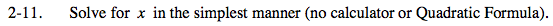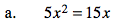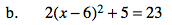### Home > PC > Chapter 2 > Lesson 2.1.1 > Problem2-11

2-11.
1. Solve for x in the simplest manner (no calculator or Quadratic Formula). Homework Help ✎1. 5x2 = 15x

2. 2(x − 6)2 + 5 = 231. Move all variables to one side equal to 0.
2. Factor.
3. Find the values of x making the equation true.1. Subtract 5 from both sides.
2. Then divide both sides by 2.
3. Take the square root of both sides.
4. Be careful with the signs!
5. Solve for values of x.